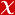## Reduced order modelling of nonlinear cross-diffusion systems

Applied Mathematics and Computation, vol.401, 2021 (Peer-Reviewed Journal)• Publication Type: Article / Article
• Volume: 401
• Publication Date: 2021
• Doi Number: 10.1016/j.amc.2021.126058
• Journal Name: Applied Mathematics and Computation
• Journal Indexes: Science Citation Index Expanded, Scopus, Academic Search Premier, Applied Science & Technology Source, Computer & Applied Sciences, INSPEC, Public Affairs Index, zbMATH, Civil Engineering Abstracts
• Keywords: Pattern formation, Finite differences, Entropy, Proper orthogonal decomposition, Principal interval decomposition, Tensor algebra

#### Abstract

© 2021 Elsevier Inc.In this work, we present reduced-order models (ROMs) for a nonlinear cross-diffusion problem from population dynamics, the Shigesada-Kawasaki-Teramoto (SKT) equation with Lotka-Volterra kinetics. The formation of the patterns of the SKT equation consists of a fast transient phase and a long stationary phase. Reduced order solutions are computed by separating the time into two time-intervals. In numerical experiments, we show for one- and two-dimensional SKT equations with pattern formation, the reduced-order solutions obtained in the time-windowed form, i.e., principal decomposition framework, are more accurate than the global proper orthogonal decomposition solutions obtained in the whole time interval. The finite-difference discretization of the SKT equation in space results in a system of linear-quadratic ordinary differential equations. The ROMs have the same linear-quadratic structure as the full order model. Using the linear-quadratic structure of the ROMs, the computation of the reduced-order solutions is further accelerated by the use of proper orthogonal decomposition in a tensorial framework so that the computations in the reduced system are independent of the full-order solutions. Furthermore, the prediction capabilities of the ROMs are illustrated for one- and two-dimensional patterns. Finally, we show that the entropy is decreasing by the reduced solutions, which is important for the global existence of solutions to the nonlinear cross-diffusion equations such as the SKT equation.